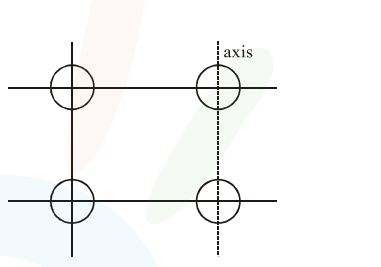# Four identical solid spheres each of mass 'm' and radius`
Question:

Four identical solid spheres each of mass 'm' and radius 'a' are placed with their centres on the four corners of a square of side ' $b$ '. The moment of inertia of the system about one side of square where the axis of rotation is parallel to the plane of the square is :

1. $\frac{4}{5} m a^{2}+2 m b^{2}$

2. $\frac{8}{5} \mathrm{ma}^{2}+\mathrm{mb}^{2}$

3. $\frac{8}{5} m a^{2}+2 m b^{2}$

4. $\frac{4}{5} \mathrm{ma}^{2}$

Correct Option: , 3

Solution:$\mathrm{I}=2 \times\left(\frac{2}{5} \mathrm{ma}^{2}\right)+2 \times\left(\frac{2}{5} \mathrm{ma}^{2}+\mathrm{mb}^{2}\right)$

$\mathrm{I}=\frac{8}{5} m \mathrm{a}^{2}+2 m b^{2}$